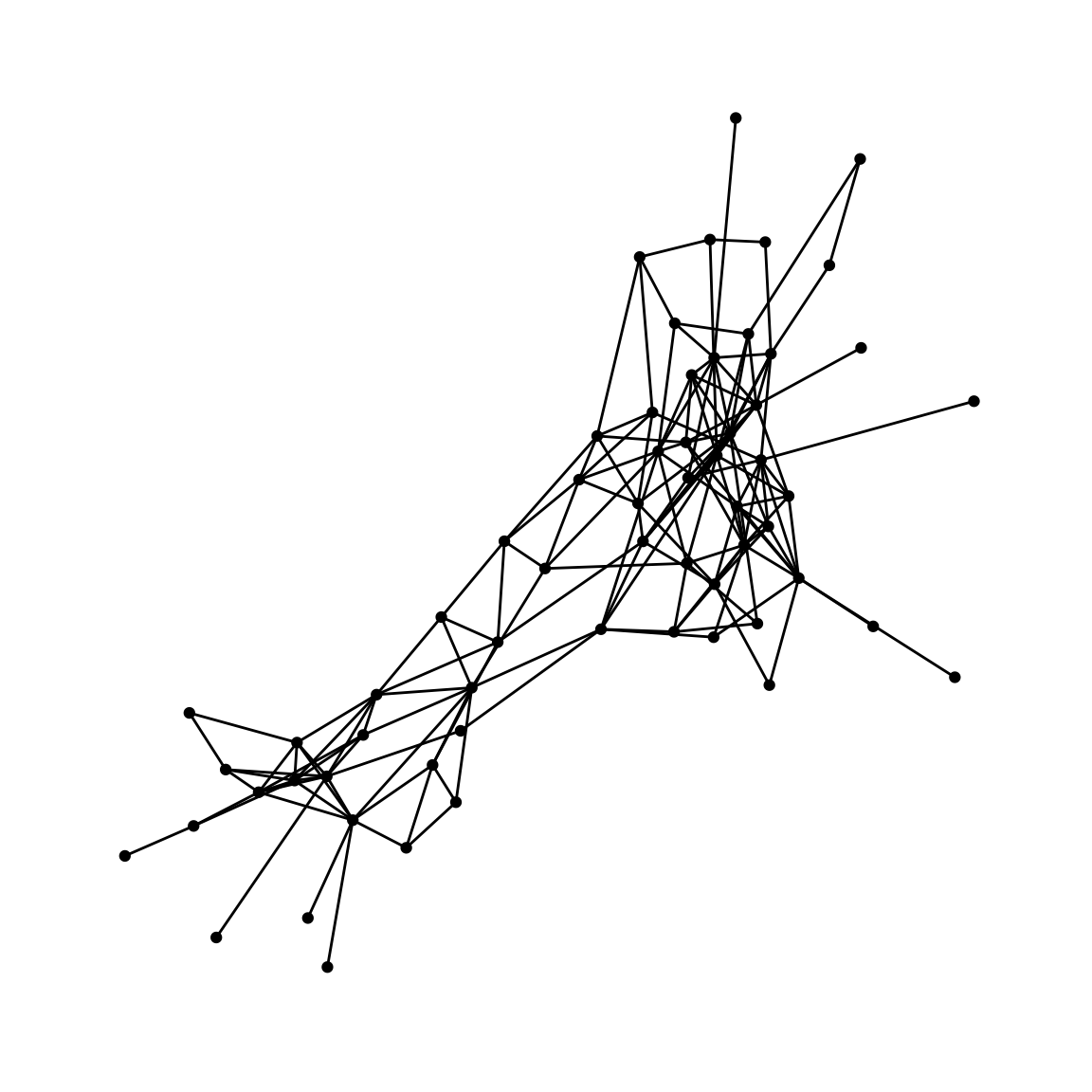``````library(readr)
library(dplyr)
library(igraph)
library(ggraph)``````

### Dataset

A social network between dolphins:

### Create graph

``````# read edges and nodes
n = nrow(nodes)
m = nrow(edges)

# mutate edges and nodes
edge_type = sample(c("love", "friendship"), m, replace = TRUE)
edge_weight = runif(m, 1, 10)
edges = mutate(edges, type = edge_type, weight = edge_weight)
nodes = mutate(nodes, id = 1:n) %>% select(id, everything())

# degree of nodes (number of ties for each dolphin)
tb = tibble(v = c(1:n, edges\$x, edges\$y))
d = count(tb, v)\$n - 1
nodes = mutate(nodes, degree = d)

# create graph from data frames
g = graph_from_data_frame(edges, directed = FALSE, nodes)``````

### Mappings

``````lay = create_layout(g, layout = "fr")

# plot with ggraph
ggraph(lay) +
geom_node_point() +
theme_graph()````````````# set theme to graph
set_graph_style()

# unset graph theme
# unset_graph_style()